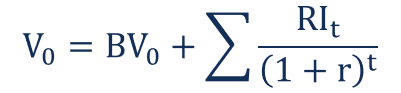# Residual Income Valuation

The value of a company’s stock equals the present value of future residual incomes discounted at the appropriate cost of equity

## What is Residual Income Valuation?

Residual income valuation (also known as residual income model or residual income method) is an equity valuation method that is based on the idea that the value of a company’s stock equals the present value of future residual incomes discounted at the appropriate cost of equity.### Further Analysis of Residual Income Valuation

The main assumption underlying residual income valuation is that the earnings generated by a company must account for the true cost of capital (i.e., both the cost of debt and cost of equity). Although the accounting for net income considers the cost of debt (interest expenses are included in the calculation of net income), it does not take into account the cost of equity since the dividends and other equity distributions are not included in the net income calculation.

Due to the above reason, the net income does not represent the company’s economic profit. Moreover, in some cases, even when a company reports accounting profits, such profits may turn out to be economically unprofitable after the consideration of equity costs.

On the other hand, residual income is the company’s income adjusted for the cost of equity. Remember that the cost of equity is essentially the required rate of return asked by investors as compensation for the opportunity cost and corresponding level of risk. Therefore, the value of a company calculated using the residual income valuation is generally more accurate since it is based on the economic profits of a company.

### Benefits of Residual Income Valuation

Generally, residual income valuation is suitable for mature companies that do not give out dividends or follow unpredictable patterns of dividend payments. In this regard, the residual income model is a viable alternative to the dividend discount model (DDM).

Additionally, it works well with companies that do not generate positive cash flows yet. However, an analyst must be aware that such an approach is based mostly on forward-looking assumptions that can be manipulated or are prone to various biases.

Along with the discounted cash flow (DCF) model, residual income valuation is one of the most recognized valuation approaches in the industry. Although the approach is less well-known, the residual income model is widely used in investment research. (Note that residual income valuation is an absolute valuation model that aims to determine a company’s intrinsic value).

### How to Calculate a Company’s Value Using the Residual Income Valuation Model?

The first step required to determine the intrinsic value of a company’s stock using residual income valuation is to calculate the future residual incomes of a company.

Recall that residual income is the net income adjusted for the cost of equity. In most cases, the residual income can be calculated as the difference between the net income and equity charge. Mathematically, it can be expressed through the following formula:

##### Residual Income = Net Income – Equity Charge

Essentially, the equity charge is a deduction from net income accounted for the cost of equity. The equity charge is a multiple of the company’s equity capital and the cost of equity capital. The formula of the equity charge is:

##### Equity Charge = Equity Capital x Cost of Equity

After the calculation of residual incomes, the intrinsic value of a stock can be determined as the sum of the current book value of the company’s equity and the present value of future residual incomes discounted at the relevant cost of equity. The valuation formula for the residual income model can be expressed in the following way:Where:

• BV– Current book value of the company’s equity
• RI– Residual income of a company at time period t
• r – Cost of equity

CFI is the official provider of the global Financial Modeling & Valuation Analyst (FMVA)™ certification program, designed to help anyone become a world-class financial analyst. To keep advancing your career, the additional resources below will be useful:

• Cost of Debt
• Dividend Payout Ratio
• Internal Rate of Return (IRR)
• Valuation Methods

### Valuation Techniques

Learn the most important valuation techniques in CFI’s Business Valuation course!

Step by step instruction on how the professionals on Wall Street value a company.

Learn valuation the easy way with templates and step by step instruction!